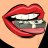# How to reference the value of a study at each bar instead of its value at the current time

L

#### lmk99

##### Member
VIP
Hi all,

I'm sure there is probably a simple answer to this but I haven't figured it out.

I am trying to paint a boolean arrow on each bar that meets the conditions of "(Variable A = Blah-blah-blah ) and (reference RSI() < 30);"

However, what is happening is the arrow only paints when the current RSI at the current time is < 30. (E.g., if it's 12pm EST and the RSI at 12PM is 56, then the arrow won't paint above that candle even though the RSI of that particular past candle was 24).

Is there a simple way to make the RSI value of each candle be used, instead of the RSI value at the current time?

PS: "GetValue" is what I needed! This is how I used it:

Code:
``````def currentRSI = getvalue(reference RSI());

def Condition1 = currentRSI  <= 18 and (Variable <= -10);
def Condition2 = currentRSI >= 70 and (Variable <= -8);``````

Last edited by a moderator:#### wtf_dude

##### Active member
Never seen somebody having to use getvalue for an RSI crossover type study. Just go ahead and post the whole code of what you're looking at?

L

#### lmk99

##### Member
VIP
Never seen somebody having to use getvalue for an RSI crossover type study. Just go ahead and post the whole code of what you're looking at?

Actually it was just example code but I was using SlowRSI, maybe that's what made it different. I fixed it though, using GetValue (at first it wouldn't work but that was because of a separate problem related to a typo on my end lol).

•wtf_dudeReference to study OR study within study Questions 14